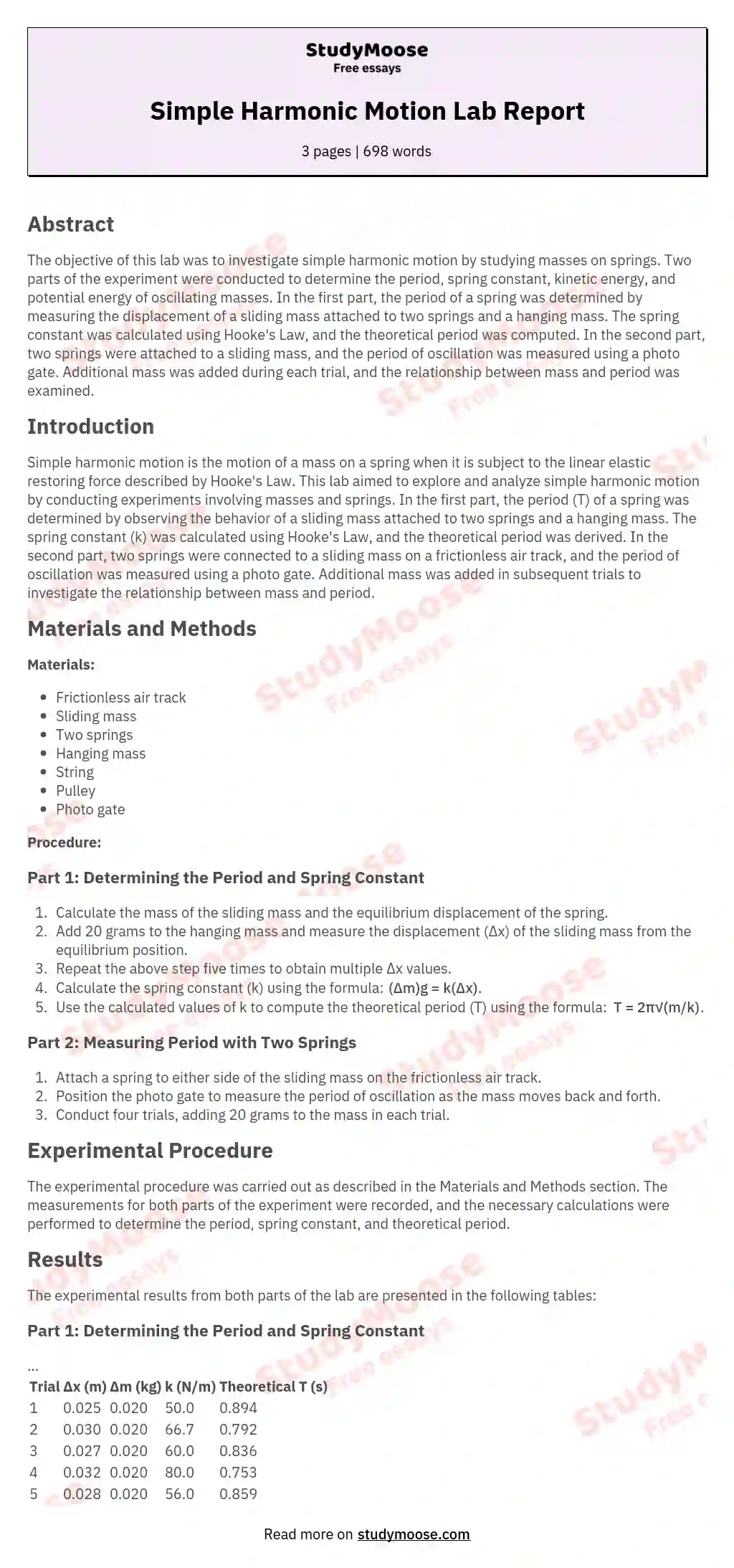# Simple Harmonic Motion Lab Report

Simple harmonic motion is the motion of a mass on a spring when it is subject to the linear elastic restoring force given by Hooke’s Law. In this lab, we will observe simple harmonic motion by studying masses on springs. In the first part of this lab, you will determine the period, T, of the spring by observing one sliding mass that is attached to two springs with the spring constant k, and attached to a hanging mass by a string and a pulley.

The next part, you will determine the period, T, of oscillation caused by two springs attached to either side of a sliding mass. By knowing the velocity in the second part, you can find kinetic energy and potential energy of the oscillating mass. Also, you must find the uncertainty in the period, kinetic energy, and potential energy. Procedure

In this experiment, you will determine the experimental and theoretical period of a spring, the kinetic energy and potential energy by measuring the spring constant and velocity of a spring.

Get quality help nowwriter-CharlotteVerified writer

Proficient in: Mechanical Engineering4.7 (348)

“ Amazing as always, gave her a week to finish a big assignment and came through way ahead of time. ”+84 relevant experts are online

In this first part of this lab, you will have a sliding mass on a frictionless air track attached to two springs on one side, and attached to a hanging mass by a string and pulley on the other. First you must calculate the mass of the sliding mass and the equilibrium displacement of the spring. The rest of the first part requires you to add 20 grams to the hanging mass and then measuring how far the sliding mass has moved for the equilibrium position.

Get to Know The Price Estimate For Your Paper
Topic
Number of pages
Email Invalid email

You won’t be charged yet!

That number will be your delta x. Do that method five times and then solve for the spring constant through the formula: (Delta m) g = k (Delta x).

The values of k that you solve for will be plugged into the formula: T = 2 (pi) (radical m/k).
The period that you solved for will be your theoretical period. In part two of this lab, you will attach a spring on either side of a sliding mass on a frictionless air track and have a photo gate measure the period as the mass oscillates. Repeat that procedure for three more times and at each trial, add 20 more grams to the mass.